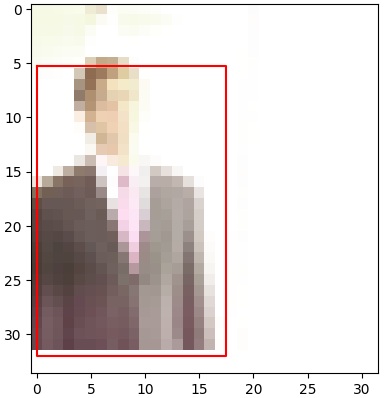# My Simplest Neural Network Object Detector Not Working Well

I made one simplest object detection task and a model to study object detection.

The task is to predict the bounding box of a person. Each image contains only one person. An image example is the following.I made the dataset from MSCOCO dataset. I chose the images containing only one person, and then resized them into (32,32). I got 1k images.

• MODEL

The SimpleObjectDetector class is made as follows:

``````class ConvLayer(nn.Module):
def __init__(self,in_channel,out_channel):
super().__init__()
self.act = nn.ReLU()
self.pool = nn.MaxPool2d(2,2)
def forward(self,x):
x = self.pool(self.act(self.conv(x)))
return x

class SimpleObjectDetector(nn.Module):
def __init__(self):
super().__init__()
self.conv1 = ConvLayer(3,8)
self.conv2 = ConvLayer(8,16)
self.l1 = nn.Linear(8*8*16,64)
self.l2 = nn.Linear(64,4)
self.act = nn.ReLU()

def forward(self,x):
x = self.conv1(x)
x = self.conv2(x)
x = x.view(x.size(),-1)
x = self.act(self.l1(x))
x = self.l2(x) * 32 + 16
return x    # (x,y,w,h) is expected.
``````

x = self.l2(x) * 32 + 16 is to make the output distribution fit the image size (32,32).

• TRAINING

I tried the loss function nn.L1Loss() and IoU loss. At 1~3 epoch, the loss stops decreasing.

• RESULT

I found the predicted bounding boxes become almost the same for all images.